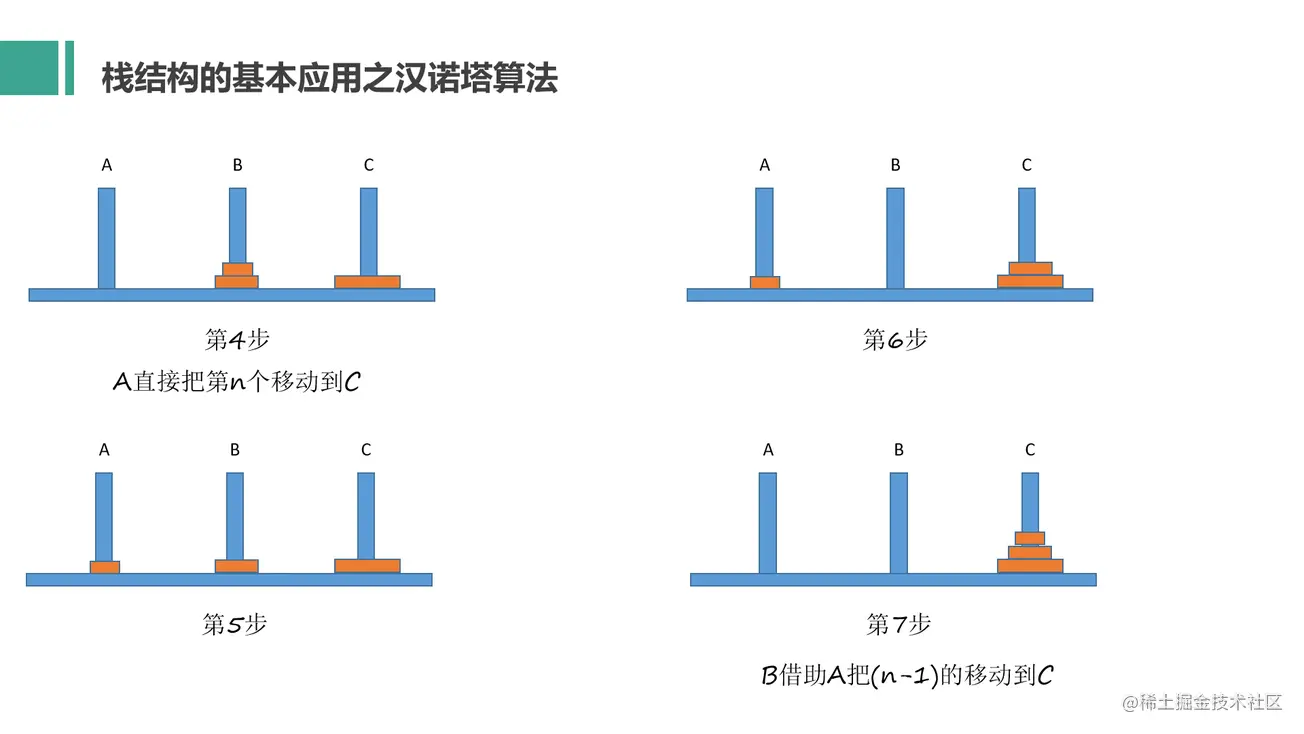# 栈的基本概念# 栈结构的自我实现

push(eles)：添加一个或者多个元素到栈顶

pop：移除当前处于栈顶的元素，同时返回被移除的元素

peek：返回当前栈顶的元素，不对栈本身进行任何修改

isEmpty：判断栈里面是否为空，如果栈里面什么也没有，就返回true，否则返回false

size：返回栈里面的元素个数，和数组的length属性类似

clear：移除栈里面的所有元素

for of（接口）：实现栈结构的迭代器接口

``````class Stack{ // 栈的类
constructor(){
this.count = 0; // 栈的计数器，用于计算栈内部有多少个元素
this.items = {}; // 栈的数据仓库，实际数据存储在这个对象中
}

push(...eles){ // 添加一个或者多个元素到栈顶
for(let i = 0, len = eles.length; i < len; i++){
this.items[this.count] = eles[i];
// 采用计数器的当前值(0到正无穷的自然数)为下标key，存储对应下标的实际数据
// this.items中不使用i而使用this.count做下标的原因是因为当下次for循环开始时，i又变成了从0开始

this.count++; // 每当push一个数据，计数器自增1
}
}

size(){ // 返回栈里面的元素个数，和数组的length属性类似
return this.count;
}

isEmpty(){ // 判断栈里面是否为空
return !this.count;
}

pop(){ // 移除当前处于栈顶的元素，同时返回被移除的元素
if(this.isEmpty){ // 有内容才需要删除
return undefined; // 如果栈内本身为空，返回undefined
}
this.count--;
// 下标是从0开始，而计数器是从1开始计数，所以计数器自减1，减去1之后得到的就是未删除前，栈顶值的key
let result = this.items[this.count]; // 用一个变量保存需要被返回栈顶的值
delete this.items[this.count]; // 删除栈顶的值
return result;
}

peek(){ // 返回当前栈顶的元素，不对栈本身进行任何修改
if(this.isEmpty()){ // 有内容才需要删除
return undefined; // 如果栈内本身为空，返回undefined
}
return this.items[this.count - 1]; // 返回当前处于栈顶的元素
}

clear(){
while(!this.isEmpty()){ // 当栈不为空时，才需要清空
this.pop() // 尽量用自己之前已经实现的方法来实现后续的其他功能
}
}

toString(){ // 把栈内所有内容变成一个字符串进行输出
if(this.isEmpty()){ // 判断是否为空
return ""; // 如果栈内本身为空，返回""
}
let resultString = ""; // 定义一个结果字符串，默认值为空字符串
for(let i = 0; i < this.count; i++){
resultString = `\${resultString},\${this.itmes[i]}`; // 每次循环都进行字符串拼接
}
return resultString.slice(1);
// 因为第一次拼接时是空字符串和元素拼接，所以需要去掉第一次拼接后留下的逗号
}

forEach(cb){ // 实现forEach接口
for(let i = 0; i < this.count; i++){
cb(i, this.items[i], this);
}
}

[Symbol.iterator](){ // 手动给栈添加迭代器接口
let self = this;
let index = 0;
return {
next(){
if(index < self.count){ // 如果当前被遍历到的元素的下标小于this.count，说明没有遍历完
return {
value: self.items[index++],
done: false
}
}else{ // 否则就是已经遍历完成
return {
value: undefined,
done: true
}
}
}
}
}
}

let arr = new Stack();
arr.push("hello") // 添加值
arr.push("world")
arr.push("你好","吃了吗")
arr.peek() // 获取栈顶值
arr.pop() // 删除一个
arr.size() // 获取长度
arr.isEmpty() // 判断是否为空
arr.toString() // 输出字符串
for(let val of arr){
console.log(val);
}
arr.forEach(function(index,item,arr){
console.log({index,item});
})
arr.clear() // 清空
``````

# 栈结构在算法中的应用

## 进制转换：

1 0 1 0 => 1×2的3次方 + 0×2的2次方 + 1×2的1次方 + 0×2的0次方 = 8 + 0 + 2 + 0 =10

10/2=5，余数是0；

5/2=2，余数是1；

2/2=1，余数是0；

1/2=0，余数是1；

``````// 导入刚刚实现的栈结构
class Stack{ // 栈的类
constructor(){
this.count = 0; // 栈的计数器，用于计算栈内部有多少个元素
this.items = {}; // 栈的数据仓库，实际数据存储在这个对象中
}

push(...eles){ // 添加一个或者多个元素到栈顶
for(let i = 0, len = eles.length; i < len; i++){
this.items[this.count] = eles[i];
// 采用计数器的当前值(0到正无穷的自然数)为下标key，存储对应下标的实际数据
// this.items中不使用i而使用this.count做下标的原因是因为当下次for循环开始时，i又变成了从0开始

this.count++; // 每当push一个数据，计数器自增1
}
}

size(){ // 返回栈里面的元素个数，和数组的length属性类似
return this.count;
}

isEmpty(){ // 判断栈里面是否为空
return !this.count;
}

pop(){ // 移除当前处于栈顶的元素，同时返回被移除的元素
if(this.isEmpty){ // 有内容才需要删除
return undefined; // 如果栈内本身为空，返回undefined
}
this.count--;
// 下标是从0开始，而计数器是从1开始计数，所以计数器自减1，减去1之后得到的就是未删除前，栈顶值的key
let result = this.items[this.count]; // 用一个变量保存需要被返回栈顶的值
delete this.items[this.count]; // 删除栈顶的值
return result;
}

peek(){ // 返回当前栈顶的元素，不对栈本身进行任何修改
if(this.isEmpty()){ // 有内容才需要删除
return undefined; // 如果栈内本身为空，返回undefined
}
return this.items[this.count - 1]; // 返回当前处于栈顶的元素
}

clear(){
while(!this.isEmpty()){ // 当栈不为空时，才需要清空
this.pop() // 尽量用自己之前已经实现的方法来实现后续的其他功能
}
}

toString(){ // 把栈内所有内容变成一个字符串进行输出
if(this.isEmpty()){ // 判断是否为空
return ""; // 如果栈内本身为空，返回""
}
let resultString = ""; // 定义一个结果字符串，默认值为空字符串
for(let i = 0; i < this.count; i++){
resultString = `\${resultString},\${this.itmes[i]}`; // 每次循环都进行字符串拼接
}
return resultString.slice(1);
// 因为第一次拼接时是空字符串和元素拼接，所以需要去掉第一次拼接后留下的逗号
}

forEach(cb){ // 实现forEach接口
for(let i = 0; i < this.count; i++){
cb(i, this.items[i], this);
}
}

[Symbol.iterator](){ // 手动给栈添加迭代器接口
let self = this;
let index = 0;
return {
next(){
if(index < self.count){ // 如果当前被遍历到的元素的下标小于this.count，说明没有遍历完
return {
value: self.items[index++],
done: false
}
}else{ // 否则就是已经遍历完成
return {
value: undefined,
done: true
}
}
}
}
}
}

// 十进制转二进制
function decimalToBinary(decNumber){
let remStack = new Stack(); // 定义一个存储余数的栈
let number = decNumber; // 存储传入的十进制参数
let rem; // 余数
let binaryString = ""; // 存储转换后的二进制数据字符串

while(number > 0){ // 只要数字还大于0，就一直除以2取余数
rem = Math.floor(number % 2); // 先取余数
remStack.push(rem); // 把余数添加到栈里面
number = Math.floor(number / 2); // 每次取余数之后，将原来的十进制数字除以2得到新的十进制数字
}

while(!remStack.isEmpty){ // 只要存储余数的栈不为空
binaryString += remStack.pop().toString(); // 取出余数，转成字符串格式后，进行拼接组合
}

return binaryString; // 最后返回
}

decimalToBinary(10); // 执行该函数
``````

## 汉诺塔：``````// 使用递归方法
function hanoi(plates, A, B, C, moves = []){ // 滑块个数，A柱，B柱，C柱，存储步骤的结果数组
if(plates <= 0){ // 柱子上没有滑块了
return moves; // 直接返回结果数组
}
if(plates === 1){ // 状态1，只有一个滑块的时候
moves.push([A,"挪到",C]); // 把A柱子的滑块移到C柱子，将此步骤存入结果数组moves

}else{ // 否则就是状态2不只一个滑块的时候，当做是一次新的移动
hanoi(plates - 1, A, C, B, moves); // 递归调用
// 除去最下面1个滑块，因为滑块要移到B柱子，所以目的地变了，传入的形参也要改变为A，C，B，
// 并且将之前存储步骤的结果数组moves添加到此次移动中，然后移动完之后此时又变成状态1

moves.push([A,"挪到",C]);
// 因为此时又是状态1，所以把A柱子的滑块移到C柱子，将此步骤存入结果数组moves

//然后此时又要开始一次新的移动，因为刚刚已经把滑块都移动到B柱子，所以此时要把B柱子上的滑块移到C柱子
hanoi(plates - 1, B, A, C, moves); // 递归调用
}
return moves; // 最后返回结果数组
}

console.log(hanoi(3,"第一根柱子","第二根柱子","第三根柱子"))
``````